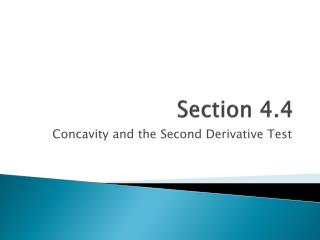DownloadDownload PresentationSection 4.4

# Section 4.4

Download Presentation## Section 4.4

- - - - - - - - - - - - - - - - - - - - - - - - - - - E N D - - - - - - - - - - - - - - - - - - - - - - - - - - -
##### Presentation Transcript

1. Section 4.4 Concavity and the Second Derivative Test

2. Concavity

3. Concavity (cont.)

4. Example 1 State the signs of and on the interval (0,2).

5. Example 2 State the signs of and on the interval (0,2).

6. Example 3 Determine the open intervals on which the graph is concave upward or concave downward.

7. Example 4 Determine the open intervals on which the graph is concave upward or concave downward.

8. Example 5 Determine the open intervals on which the graph is concave upward or concave downward.

9. Inflection Points • Examples:

10. How do we find them? • So . . . • Take the second derivative. • Find out where it is zero or undefined. • These are your possible inflection points. • Only an inflection point if the concavity changes.

11. Example 6 Find the points of inflection and discuss the concavity of the graph of the function.

12. Example 7 Find the points of inflection and discuss the concavity of the graph of the function.

13. Example 8 Find the points of inflection and discuss the concavity of the graph of the function.

14. The Second Derivative Test

15. Example 9 Find all relative extrema. Use the Second Derivative Test where applicable.

16. Example 10 Find all relative extrema. Use the Second Derivative Test where applicable.

17. Example 11 Find all relative extrema. Use the Second Derivative Test where applicable.

18. Questions? Be sure to be practicing the given problem sets!